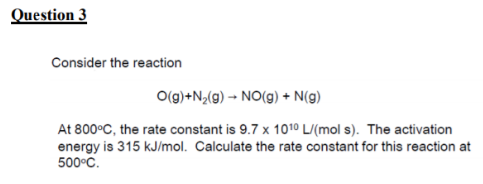# Problem: Consider the reaction O (g) + N2 (g) → NO (g) + N (g) At 800°C, the rate constant is 9.7 x 1010 L/(mol s). The activation energy is 315 kJ/mol. Calculate the rate constant for this reaction at 500°C.

###### FREE Expert Solution
90% (236 ratings)###### Problem Details

Consider the reaction

O (g) + N2 (g) → NO (g) + N (g)

At 800°C, the rate constant is 9.7 x 1010 L/(mol s). The activation energy is 315 kJ/mol. Calculate the rate constant for this reaction at 500°C.